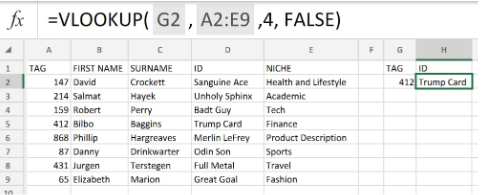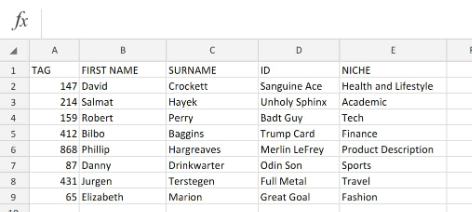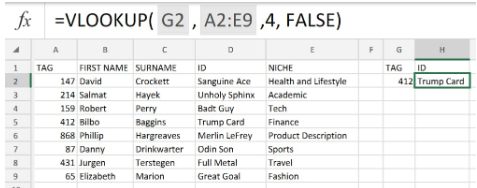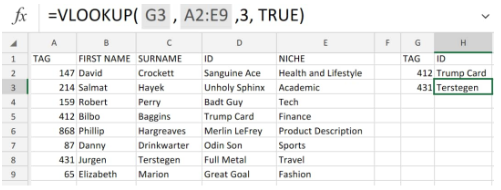Get instant live expert help with Excel or Google Sheets“My Excelchat expert helped me in less than 20 minutes, saving me what would have been 5 hours of work!”

#### Post your problem and you’ll get expert help in seconds.

Your message must be at least 40 characters
Our professional experts are available now. Your privacy is guaranteed.

# How to troubleshoot VLOOKUP issues – Excelchat

We can Use the  VLOOKUP function in Excel to locate and retrieve data from a work table column or row.

Excel VLOOKUP is categorized as a  lookup and reference tool.Figure 1. of VLOOKUP in Excel

## Generic Formula

`=VLOOKUP(value,table,col_index,[range_lookup])`

• Value = The value to be looked for in the initial column of a work table.
• Table = The entire cells from which we are to retrieve a value.
• Col_index = The column number in the work table from which we are to retrieve a value.
• Range_lookup (optional) = Enter FALSE for an exact match; TRUE (default) for an approximate match.

## How to Use the VLOOKUP Function in Excel

VLOOKUP Excel is designed to locate and recover data from worksheet data which has been entered into vertical columns and horizontal rows; each row is representing a new entry.

The “V” in “VLOOKUP” means “Vertical”.

We will now show you how to use the VLOOKUP Function in Excel:

1. Arrange the data available to us in vertical work sheet columns:Figure 2. of VLOOKUP Data in Excel

The VLOOKUP Excel Function only looks to the right; this means that VLOOKUP requires our lookup table to be set-up with lookup values contained in the extreme left column.

The data we want to retrieve (our result values) may appear inside any column on the right-hand side.

1. In the worksheet example above (Figure 1.) our goal is to make use of Excel VLOOKUP to check the cells in column A (TAG) for a specified value and retrieve an exact matching value from the worktable.

To do this we will enter the following VLOOKUP formula into cell H2 of our worksheet example;

`=VLOOKUP(G2,A2:E9,4,FALSE)`Figure 3. of VLOOKUP in Excel

Because VLOOKUP Excel retrieves data based a given column number, whenever we use VLOOKUP, just imagine that each column on our table is numbered, beginning from the left side.

To retrieve a particular value from an Excel column, just supply the appropriate column number in the Excel VLOOKUP formula:

`=VLOOKUP(G3,A2:E9,3,TRUE`Figure 4. of VLOOKUP in Excel

## VLOOKUP TROUBLESHOOTING

Even though VLOOKUP Excel is considered to be a very intricate Excel function, it still has lots of limitations, which can cause many problems and errors.

We will now highlight the most frequent VLOOKUP issues and most the obvious reasons why Excel vlookup is not working.

VLOOKUP not returning correct value – Vlookup formulas will display a #N/A (not available) error message when Excel cannot locate a given lookup value. There are several reasons for this happening:

• #### Extra spaces existing inside our lookup column